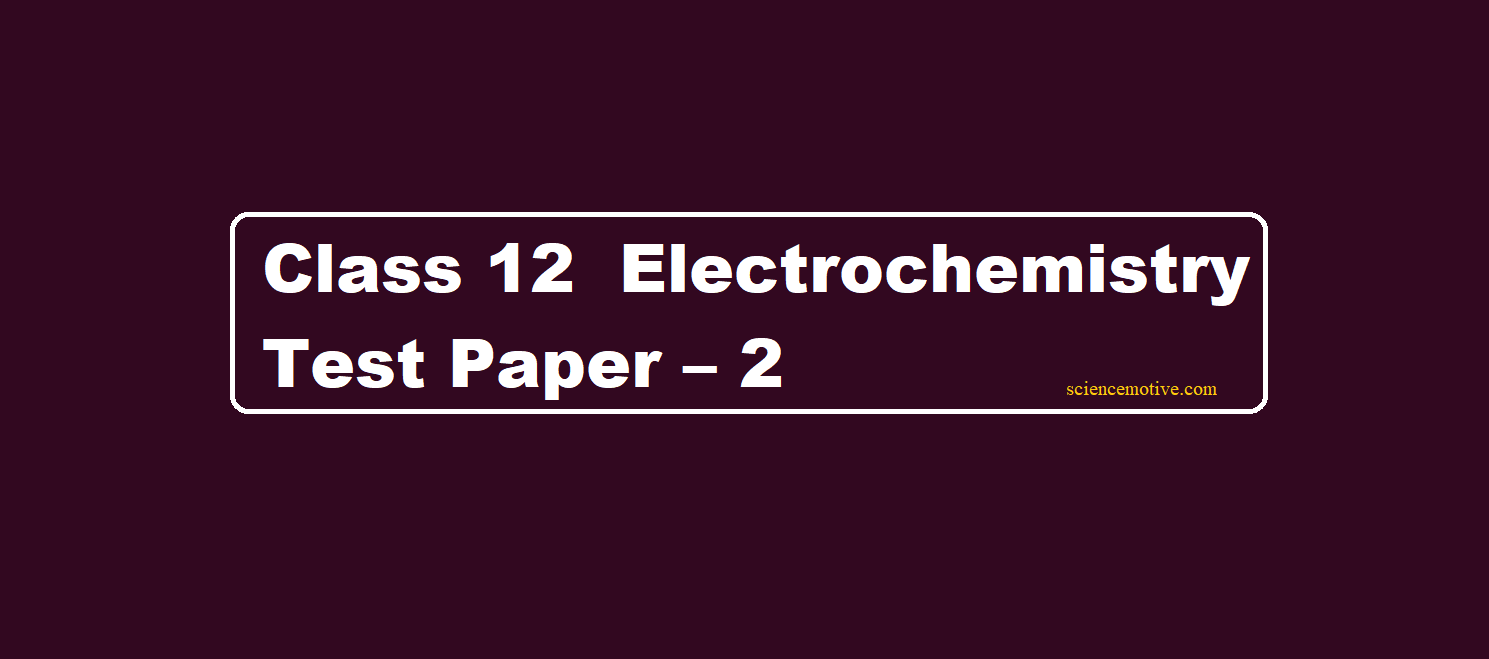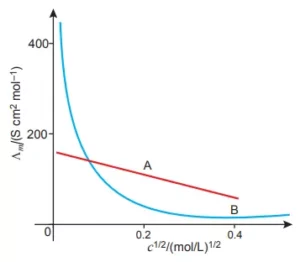# Class 12 Electrochemistry Test Paper – 2# Class 12 Electrochemistry Test Paper – 2

Class 12 Electrochemistry Test Paper

Que 1. What is an electrochemical series? How does it predict the feasibility of a specific redox reaction?

Que 2. Give some uses of electrochemical cells?

Que 3. How is the standard electrode potential of a cell related to:
(a) Equilibrium constant?
(b) Gibbs free energy change

Que 4. What are the factors on which the conductivity of an electrolyte depends?

Que 5. State Kohlrausch law. Write the reaction which occurs at the electrode of the hydrogen-oxygen fuel cell.

Que 6. A current of 2A is passed for 1 hr between Ni electrodes in 0.5 L of 2M Ni (NO3)2 solution. What will be the Molarity of sol at the end of electrolysis?

Que 7. Name the factors on which Metallic and Ionic Conductance depend upon?

Que 8. One half-cell in a voltaic cell is constructed from a silver wire dipped in a silver nitrate solution of unknown concentration. The other half-cell consists of a zinc electrode in a 0.10 M solution of Zn(NO3)2. A voltage of 1.48 V is measured for this cell. Use this information to calculate the concentration of silver ions in the solution. (Given: E0Zn2+/ Zn = – 0.763 V, E0Ag+/Ag = + 0.80 V)

Que 9. Explain the construction and working of standard Hydrogen electrodes?

Que 10. A cell is represented by notation: Cu (s) /cu2+ (aq) // Ag+ (aq) / Ag (s). Calculate e.m.f of the cell if E0Cu2+/Cu = + 0.34V and E0Ag+/Ag = 0.80V?

Que 11. At infinite dilution, the molar conductance of Na+ and SO42- ions are 50 and 160 S cm2 mol-1 respectively. What will be the molar conductance of sodium sulphate at infinite dilution?

Que 12. Predict the products of electrolysis obtained at the electrodes in each case when the electrodes used are platinum.
(a) An aqueous solution of AgNO3
(b) A dilute aqueous solution of H2SO4

Que 13. Ag is electro-deposited on a metallic vessel of surface area of 800 cm2 by passing a current of 0.2 A for 3 hrs. Calculate the thickness of Ag deposited. Density of Ag = 10.47 g/cc At. Mass Ag = 107.92 amu.

Que 14. The measured resistance of a cell containing 7.5 × 10-3 M solution of KCl at 25 0C was 1005 Ω calculate (a) Specific conductance and
(b) Molar conductance of the solution.
Cell Constant = 1.25 cm-1 A, k = 1.243 × 10-3 Ω-1 cm-1 λm = 165.7 W-1cm2 mol-1.

Que 15. The conductivity of an aqueous solution of NaCl in a cell is 92 Ω-1 cm-1. The resistance offered by this cell is 247.8 Ω. Calculate the cell constant?

Que 16. For what concentration of Ag+ (aq) will e.m.f of the cell be zero at 25 0C if concentration of Cu2+ 0.01 M? Cu|Cu2+ (0.01M) || Ag+ (aq) | Ag(s)  Given: E0Ag+ /Ag = + 0.80 V and E0Cu2+/Cu = + 0.34 V.

Que 17. The half-cell reaction are
(i) Fe3+ + e → Fe2+: E0 = 0.76V
(ii) Ag+ (aq) + 1e → Ag (s): E0 = 0.80 V
Calculate Kc for the following reaction at 25 0C. Ag+ (aq) + Fe2+ → Ag (S) + Fe3+ Given 1F = 96500 C mol-1.

Que 18. Silver is electrodeposited on a metallic vessel with a total surface area of 900 cm2 bypassing a current of 0.5 A for 2 hours. Calculate the thickness of silver deposited. [Given: density of silver = 10.5 g cm-3, At. Mass of Ag=108 amu, F = 96500 C mol-1].

Que 19. What would happen if no salt bridge were used in an electrochemical cell (like Zn–Cu cell)?

Que 20. The following curve is obtained when molar conductivity (λm) is plotted against the square root of concentration, c1/2 for two electrolytes A and B.
(i) How do you account for the increase in the molar conductivity of the electrolyte A on dilution?
(ii) As seen from the graph, the value of limiting molar conductivity (λom) for electrolyte B cannot be obtained graphically. How can this value be obtained?Class 12 Electrochemistry Test Paper